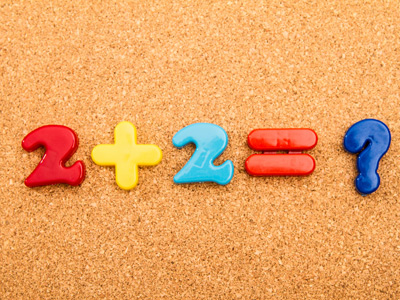In maths, a sum is the result of adding two or more numbers.

# Following Instructions 1

This is the first in our series of Eleven Plus Verbal Reasoning quizzes on Following Instructions. I’m sure you know how to follow written instructions, but these questions are a little different to the norm. In them, you will be following instructions to do with figures, or numbers.

You would be forgiven for thinking that these quizzes belong in our maths section, rather than our verbal reasoning one. Rest assured, they are in the right place. Sometimes, the 11+ Verbal Reasoning paper will include questions like these, to test your mathematical vocabulary.

If you already know the meaning of words associated with maths, like product, sum, dividend and divisor, you’ll sail through these quizzes. If not, you will learn some new things essential for your success. Good luck!

Example:
Multiply the first figure by the sum of the last two:
8, 5, 4

The sum of the last two digits is 9 (5 + 4 = 9). The answer is 9 x 8 = 72
1.
Add the first figure to the product of the last two:
5, 2, 7
19
14
24
70
The product of the last two digits is 14 (2 x 7 = 14). The answer is 5 + 14 = 19
2.
Multiply the second figure by the sum of the first and last:
4, 6, 2
48
36
24
12
The sum of the first and last figures is 6 (4 + 2 = 6). The answer is 6 x 6 = 36
3.
Multiply the first figure by the sum of the last two:
3, 4, 8
56
26
36
16
The sum of the last two digits is 12 (4 + 8 = 12). The answer is 3 x 12 = 36
4.
Subtract the first figure from the second, then multiply your answer by the third:
18, 32, 3
14
-42
28
42
The second figure minus the first is 14 (32 - 18 = 14). The answer is 14 x 3 = 42
5.
Add the last figure to the product of the first two:
2, 8, 7
17
23
58
37
The product of the first two figures is 16 (2 x 8 = 16). The answer is 16 + 7 = 23
6.
Subtract the first figure from the product of the last two:
30, 8, 9
42
-13
-42
13
The product of the last two figures is 72 (8 x 9 = 72). The answer is 72 - 30 = 42
7.
Add the first figure to the quotient of the last two (the middle figure is the dividend and the last is the divisor):
17, 36, 12
20
29
19
65
The quotient of the last two figures is 3, if 36 is the dividend and 12 the divisor (36 ÷ 12 = 3). The answer is 17 + 3 = 20
8.
Subtract the first figure from the quotient of the last two (the last figure is the dividend and the middle one is the divisor):
5, 3, 33
94
16
6
-2
The quotient of the last two figures is 11, if 33 is the dividend and 3 the divisor (33 ÷ 3 = 11). The answer is 11 - 5 = 6
9.
Multiply the last figure by the sum of the first two:
4, 9, 2
44
72
18
26
The sum of the first two figures is 13 (4 + 9 = 13). The answer is 13 x 2 = 26
10.
Subtract the third figure from the quotient of the first two (the first figure is the dividend and the second is the divisor):
15, 3, 1
17
44
14
4
The quotient of the first two figures is 5, if 15 is the dividend and 3 the divisor (15 ÷ 3 = 5). The answer is 5 - 1 = 4
Author:  Stephen O'Hara and Ian Miles